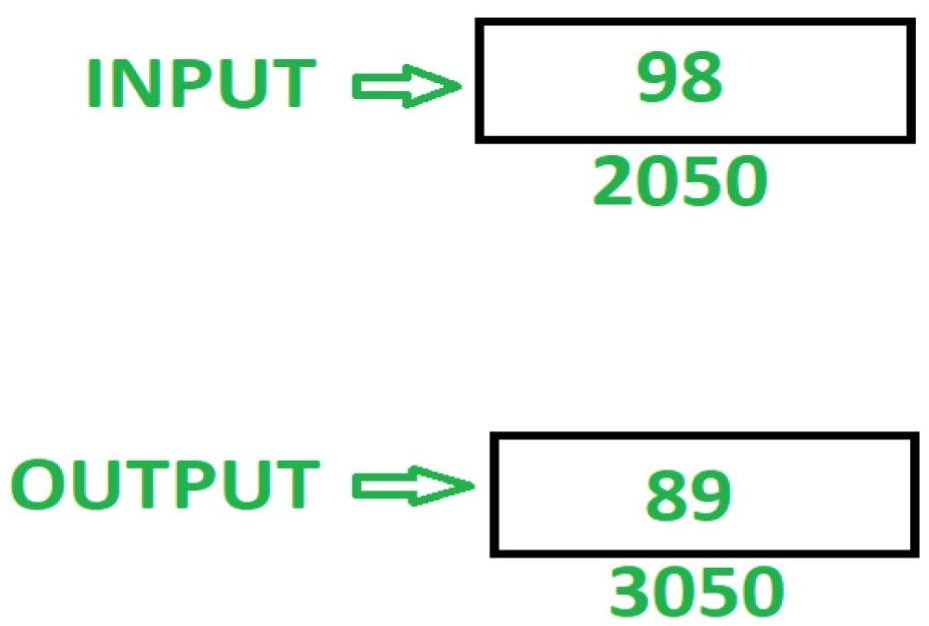GFG App
Open AppBrowser
Continue

# 8085 program to reverse 8 bit number

Problem: Write an assembly language program in 8085 microprocessor to reverse 8-bit numbers.

Example:Assume that number to be reversed is stored at memory location 2050, and reversed number is stored at memory location 3050.

Algorithm –

1. Load content of memory location 2050 in accumulator A
2. Use RLC instruction to shift the content of A by 1 bit without carry. Use this instruction 4 times to reverse the content of A
3. Store content of A in memory location 3050

Program –

Explanation – Register A used:

1. LDA 2050: load value of memory location 2050 in Accumulator A.
2. RLC: Rotate content of accumulator left by 1 bit
3. RLC: Rotate content of accumulator left by 1 bit
4. RLC: Rotate content of accumulator left by 1 bit
5. RLC: Rotate content of accumulator left by 1 bit
6. STA 3050: store content of A in memory location 3050.
7. HLT: stops executing the program and halts any further execution.

Another Alternative and simple way to execute the above program and the magic of 8085 rotate instructions :

This can be done by using 4 times RRC instruction.

LDA 2050H

RRC

RRC              // 4 RRC do same work as 4 RLC. So we can use anyone alternatively.

RRC

RRC             //After this 4th RRC instruction our 8 bit number is reversed and stored in accumulator.

STA 3050H

HLT

Step-by-Step and Bit-by-Bit explanation of the Above Program with an example :

```Example :
98H in Binary Written as :
1001 1000
RLC 1st Time : 0011 0001  {Carry Flag = 1}
RLC 2nd Time : 0110 0010  {Carry Flag = 0}
RLC 3rd Time : 1100 0100  {Carry Flag = 0}
RLC 4th Time : 1000 1001 { Carry Flag = 1}
Converted Number after 4th RLC : 1000 1001 [89H]
Hence our number is reversed from 98H to 89H.

For Example : 98H in Binary Written as :
1001 1000
RRC 1st Time : 0100 1100  {Carry Flag = 0}
RRC 2nd Time : 0010 0110  {Carry Flag = 0}
RRC 3rd Time : 0001 0011 { Carry Flag = 0}
RRC 4th Time : 1000 1001 { Carry Flag = 1}
Converted Number after 4th RRC : 1000 1001 [89H]
Hence our number is reversed from 98H to 89H.
Hence Instead of 4 RLC, we can also use 4 RRC instructions in our code alternatively.```
My Personal Notes arrow_drop_up